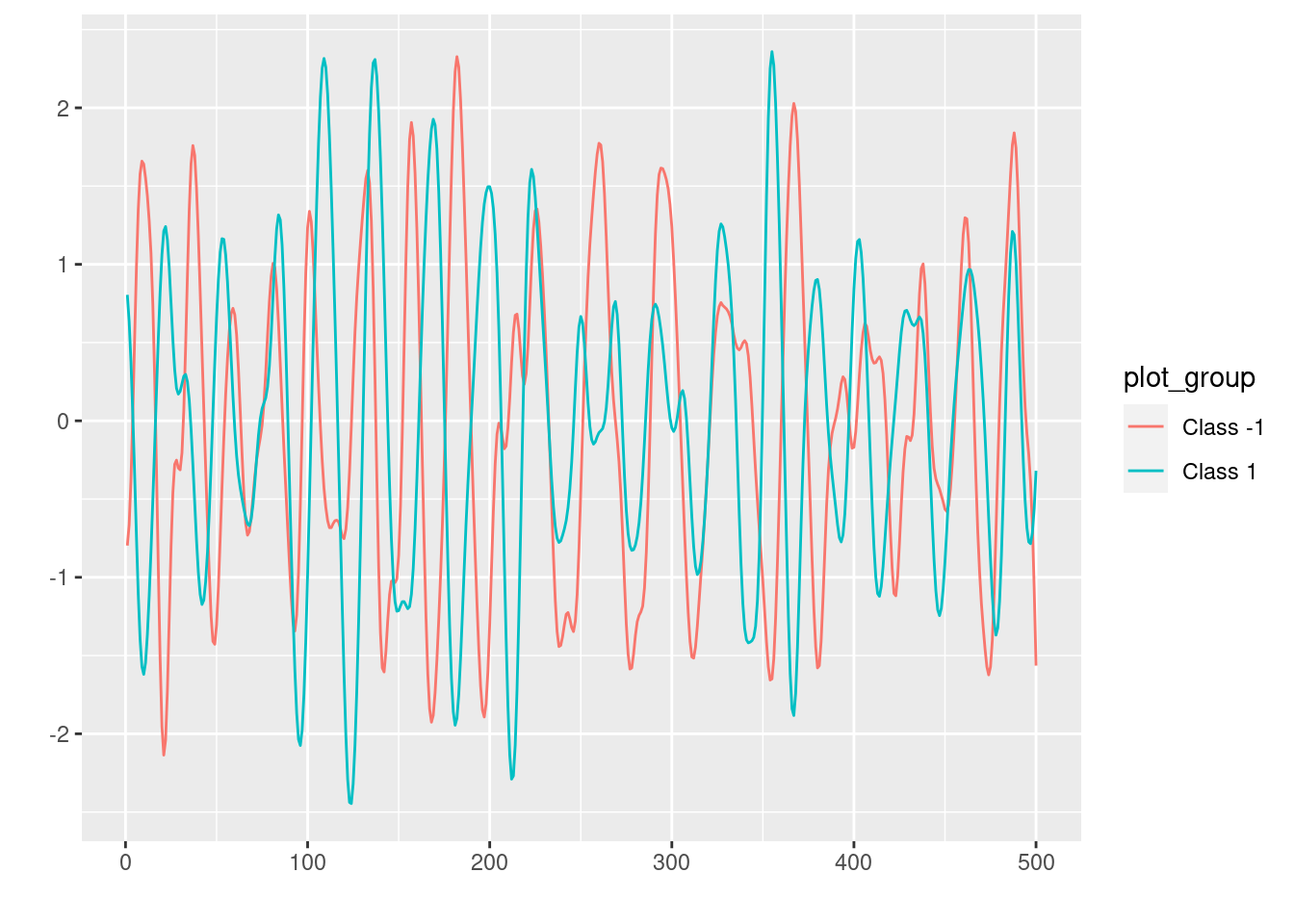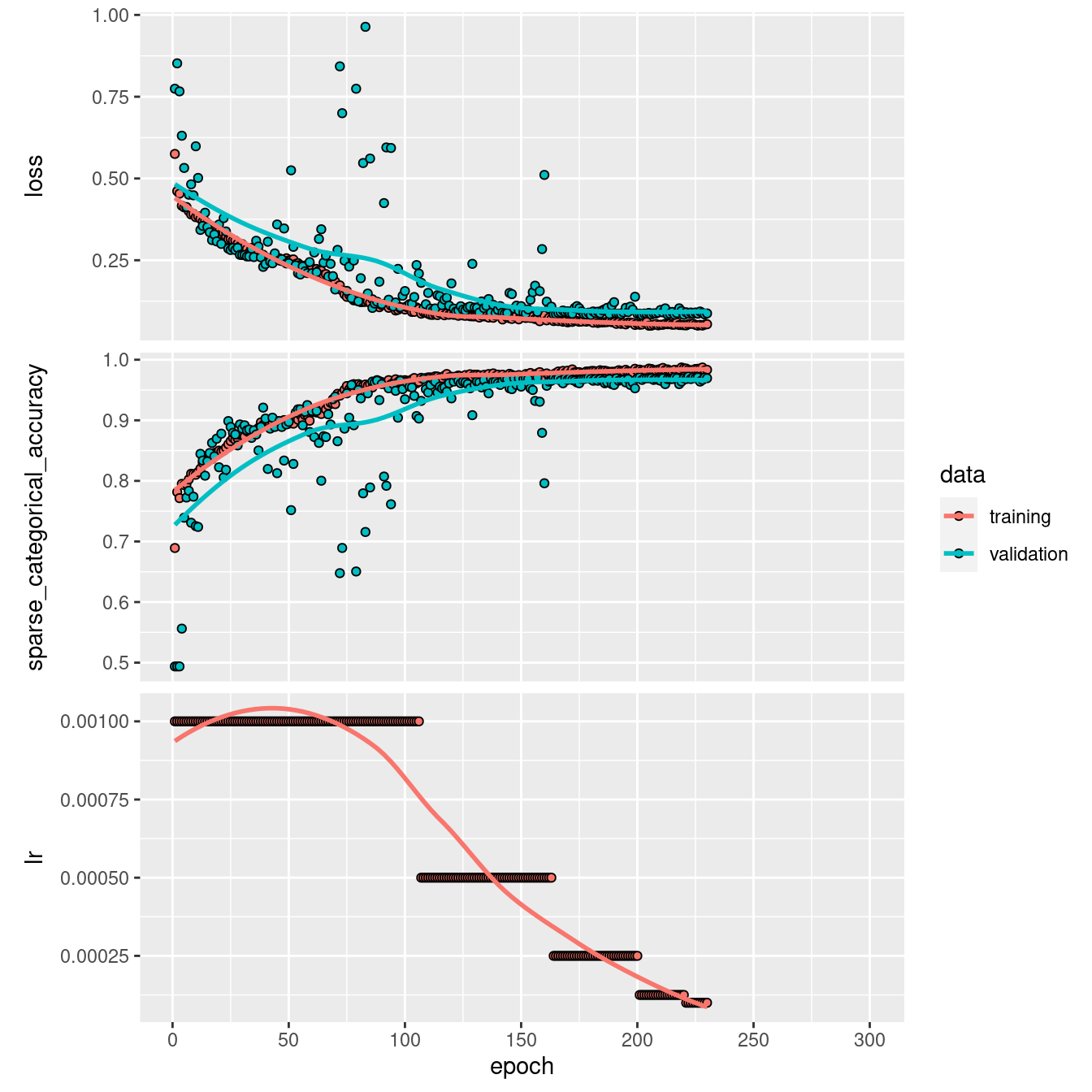# Timeseries classification from scratch

timeseries
Training a timeseries classifier from scratch on the FordA dataset from the UCR/UEA archive.
Authors

## Introduction

This example shows how to do timeseries classification from scratch, starting from raw CSV timeseries files on disk. We demonstrate the workflow on the FordA dataset from the UCR/UEA archive.

## Setup

``````library(tensorflow)
library(keras)
set.seed(1234)``````
``````url <- "https://raw.githubusercontent.com/hfawaz/cd-diagram/master/FordA"

train_df <- "FordA_TRAIN.tsv" %>%
get_file(., file.path(url, .)) %>%
x_train <- as.matrix(train_df[, -1])
y_train <- as.matrix(train_df[, 1])

test_df <- "FordA_TEST.tsv" %>%
get_file(., file.path(url, .)) %>%
x_test <- as.matrix(test_df[, -1])
y_test <- as.matrix(test_df[, 1])``````

## Visualize the data

Here we visualize one timeseries example for each class in the dataset.

``library(ggfortify)``
``Loading required package: ggplot2``
``````autoplot(ts(tibble::tibble(
"Class -1" = x_train[1, ],
"Class 1" = x_train[2, ]
)), ts.geom = 'line', facets = FALSE)``````## Standardize the data

Our timeseries are already in a single length (500). However, their values are usually in various ranges. This is not ideal for a neural network; in general we should seek to make the input values normalized. For this specific dataset, the data is already z-normalized: each timeseries sample has a mean equal to zero and a standard deviation equal to one. This type of normalization is very common for timeseries classification problems, see Bagnall et al. (2016).

In order to use `sparse_categorical_crossentropy`, we will have to count the number of classes beforehand.

``(num_classes <- length(unique(y_train)))``
`` 2``

Now we shuffle the training set because we will be using the `validation_split` option later when training.

``````shuffle_ind <- sample(nrow(x_train))
x_train <- x_train[shuffle_ind, ]
y_train <- y_train[shuffle_ind, ]``````

Standardize the labels to positive integers. The expected labels will then be 0 and 1.

``````y_train[y_train == -1] <- 0
y_test[y_test == -1] <- 0``````

Note that the timeseries data used here are univariate, meaning we only have one channel per timeseries example. We will therefore transform the timeseries into a multivariate one with one channel using a simple reshaping. This will allow us to construct a model that is easily applicable to multivariate time series.

``````# add channel dim of size 1
dim(x_train) <- c(dim(x_train), 1)
dim(x_test) <- c(dim(x_test), 1)``````

## Build a model

We build a Fully Convolutional Neural Network originally proposed in this paper. The implementation is based on the TF 2 version provided here. The following hyperparameters (kernel_size, filters, the usage of BatchNorm) were found via random search using KerasTuner.

``````input_shape <- dim(x_train)[-1] # drop batch dim
input_layer <- layer_input(input_shape)

output_layer <- input_layer %>%

# First convolutional layer
layer_conv_1d(64, 3, padding = "same") %>%
layer_batch_normalization() %>%
layer_activation_relu() %>%

# Second convolutional layer
layer_conv_1d(64, 3, padding = "same") %>%
layer_batch_normalization() %>%
layer_activation_relu() %>%

# Third convolutional layer
layer_conv_1d(64, 3, padding = "same") %>%
layer_batch_normalization() %>%
layer_activation_relu() %>%

layer_global_average_pooling_1d() %>%
layer_dense(num_classes, activation = "softmax")

model <- keras_model(input_layer, output_layer)``````

## Train the model

``````epochs <- 300
batch_size <- 32
callbacks <- list(
callback_model_checkpoint("best_model.h5", monitor = "val_loss",
save_best_only = TRUE),
callback_reduce_lr_on_plateau(monitor = "val_loss", factor = 0.5,
patience = 20, min_lr = 0.0001),
callback_early_stopping(monitor = "val_loss", patience = 50,
verbose = 1)
)

model %>% compile(
loss = "sparse_categorical_crossentropy",
metrics = list("sparse_categorical_accuracy")
)

history <- model %>%
fit(x_train, y_train,
batch_size = batch_size,
epochs = epochs,
callbacks = callbacks,
validation_split = 0.2,
verbose = 1)``````

## Evaluate model on test data

``````loaded_model <- load_model_hdf5("best_model.h5")
result <- loaded_model %>% evaluate(x_test, as.matrix(y_test))

sprintf("Test loss: %s", result[["loss"]])``````
`` "Test loss: 0.103852786123753"``
``sprintf("Test accuracy: %s", result[["sparse_categorical_accuracy"]])``
`` "Test accuracy: 0.966666638851166"``

## Plot the model’s training and validation loss

``plot(history)``We can see how the training accuracy reaches almost 0.95 after 100 epochs. However, by observing the validation accuracy we can see how the network still needs training until it reaches almost 0.97 for both the validation and the training accuracy after 200 epochs. Beyond the 200th epoch, if we continue on training, the validation accuracy will start decreasing while the training accuracy will continue on increasing: the model starts overfitting.